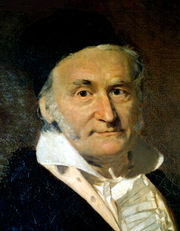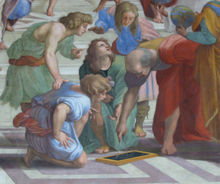## Monday, November 27, 2006

### Mathematics as scienceCarl Friedrich Gauss referred to mathematics as "the Queen of the Sciences". In the original Latin Regina Scientiarum, as well as in German Königin der Wissenschaften, the word corresponding to science means (field of) knowledge. Indeed, this is also the original meaning in English, and there is no doubt that mathematics is in this sense a science. The specialization restricting the meaning to natural science is of later date. If one considers science to be strictly about the physical world, then mathematics, or at least pure mathematics, is not a science. Albert Einstein has stated that "as far as the laws of mathematics refer to reality, they are not certain; and as far as they are certain, they do not refer to reality."

Many philosophers believe that mathematics is not experimentally falsifiable,[citation needed] and thus not a science according to the definition of Karl Popper. However, in the 1930s important work in mathematical logic showed that mathematics cannot be reduced to logic, and Karl Popper concluded that "most mathematical theories are, like those of physics and biology, hypothetico-deductive: pure mathematics therefore turns out to be much closer to the natural sciences whose hypotheses are conjectures, than it seemed even recently." Other thinkers, notably Imre Lakatos, have applied a version of falsificationism to mathematics itself.

An alternative view is that certain scientific fields (such as theoretical physics) are mathematics with axioms that are intended to correspond to reality. In fact, the theoretical physicist, J. M. Ziman, proposed that science is public knowledge and thus includes mathematics. In any case, mathematics shares much in common with many fields in the physical sciences, notably the exploration of the logical consequences of assumptions. Intuition and experimentation also play a role in the formulation of conjectures in both mathematics and the (other) sciences. Experimental mathematics continues to grow in importance within mathematics, and computation and simulation are playing an increasing role in both the sciences and mathematics, weakening the objection that mathematics does not use the scientific method. In his 2002 book A New Kind of Science, Stephen Wolfram argues that computational mathematics deserves to be explored empirically as a scientific field in its own right.

The opinions of mathematicians on this matter are varied. While some in applied mathematics feel that they are scientists, those in pure mathematics often feel that they are working in an area more akin to logic and that they are, hence, fundamentally philosophers. Many mathematicians feel that to call their area a science is to downplay the importance of its aesthetic side, and its history in the traditional seven liberal arts; others feel that to ignore its connection to the sciences is to turn a blind eye to the fact that the interface between mathematics and its applications in science and engineering has driven much development in mathematics. One way this difference of viewpoint plays out is in the philosophical debate as to whether mathematics is created (as in art) or discovered (as in science). It is common to see universities divided into sections that include a division of Science and Mathematics, indicating that the fields are seen as being allied but that they do not coincide. In practice, mathematicians are typically grouped with scientists at the gross level but separated at finer levels. This is one of many issues considered in the philosophy of mathematics.

Mathematical awards are generally kept separate from their equivalents in science. The most prestigious award in mathematics is the Fields Medal, established in 1936 and now awarded every 4 years. It is usually considered the equivalent of science's Nobel prize. Another major international award, the Abel Prize, was introduced in 2003. Both of these are awarded for a particular body of work, either innovation in a new area of mathematics or resolution of an outstanding problem in an established field. A famous list of 23 such open problems, called "Hilbert's problems", was compiled in 1900 by German mathematician David Hilbert. This list achieved great celebrity among mathematicians, and at least nine of the problems have now been solved. A new list of seven important problems, titled the "Millennium Prize Problems", was published in 2000. Solution of each of these problems carries a \$1 million reward, and only one (the Riemann hypothesis) is duplicated in Hilbert's problems.

### MathematicsMathematics (colloquially, maths, or math in American English) is the body of knowledge centered on concepts such as quantity, structure, space, and change, and the academic discipline which studies them; Benjamin Peirce called it "the science that draws necessary conclusions". It evolved, through the use of abstraction and logical reasoning, from counting, calculation, measurement, and the study of the shapes and motions of physical objects. Mathematicians explore such concepts, aiming to formulate new conjectures and establish their truth by rigorous deduction from appropriately chosen axioms and definitions.

Knowledge and use of basic mathematics have always been an inherent and integral part of individual and group life. Refinements of the basic ideas are visible in ancient mathematical texts originating in ancient Egypt, Mesopotamia, Ancient India, and Ancient China with increased rigour later introduced by the ancient Greeks. From this point on, the development continued in short bursts until the Renaissance period of the 16th century where mathematical innovations interacted with new scientific discoveries leading to an acceleration in understanding that continues to the present day.

Today, mathematics is used throughout the world in many fields, including science, engineering, medicine and economics. The application of mathematics to such fields, often dubbed applied mathematics, inspires and makes use of new mathematical discoveries and has sometimes led to the development of entirely new disciplines. Mathematicians also engage in pure mathematics for its own sake without having any practical application in mind, although applications for what begins as pure mathematics are often discovered later